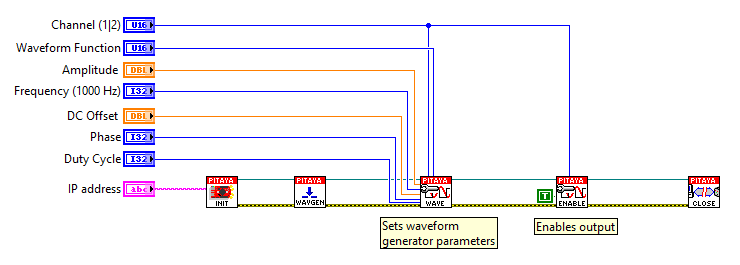# 2.3.1.4.3.1. Generate continuous signal¶

## 2.3.1.4.3.1.1. Description¶

This example shows how to program Red Pitaya to generate an analog 2 kHz sine wave signal with a 1 V amplitude. Voltage and frequency ranges depend on the Red Pitaya model.

## 2.3.1.4.3.1.2. Required hardware¶

• Red Pitaya device## 2.3.1.4.3.1.3. Code - MATLAB®¶

The code is written in MATLAB. In the code, we use SCPI commands and TCP client communication. Copy the code from below into the MATLAB editor, save the project, and hit the “Run” button.

```%% Define Red Pitaya as TCP client object

IP = '192.168.178.111';           % Input IP of your Red Pitaya...
port = 5000;
RP = tcpclient(IP, port);

%% Open connection with your Red Pitaya

RP.ByteOrder = "big-endian";
configureTerminator(RP, "CR/LF");

writeline(RP,'GEN:RST');                    % Reset Generator
writeline(RP,'SOUR1:FUNC SINE');            % Set function of output signal
% {sine, square, triangle, sawu,sawd, pwm}
writeline(RP,'SOUR1:FREQ:FIX 2000');        % Set frequency of output signal
writeline(RP,'SOUR1:VOLT 1');               % Set amplitude of output signal
writeline(RP,'OUTPUT1:STATE ON');           % Set output to ON

writeline(RP,'SOUR1:TRIG:INT');             % Generate trigger

%% Close connection with Red Pitaya

clear RP;
```

## 2.3.1.4.3.1.4. Code - C¶

Note

Although the C code examples don’t require the use of the SCPI server, we have included them here to demonstrate how the same functionality can be achieved with different programming languages. Instructions on how to compile the code are here.

```/* Red Pitaya C API example Generating continuous signal
* This application generates a specific signal */

#include <stdio.h>
#include <stdint.h>
#include <stdlib.h>
#include <unistd.h>

#include "rp.h"

int main(int argc, char **argv){

/* Print error, if rp_Init() function failed */
if(rp_Init() != RP_OK){
fprintf(stderr, "Rp api init failed!\n");
}

/* Reset generator */
rp_GenReset();

/* Generating wave form */
rp_GenWaveform(RP_CH_1, RP_WAVEFORM_SINE);

/* Generating frequency */
rp_GenFreq(RP_CH_1, 2000.0);

/* Generating amplitude */
rp_GenAmp(RP_CH_1, 1.0);

/* Enable channel */
rp_GenOutEnable(RP_CH_1);

/* Generating trigger */
rp_GenTriggerOnly(RP_CH_1);

/* Releasing resources */
rp_Release();

return 0;
}
```

## 2.3.1.4.3.1.5. Code - Python¶

Using just SCPI commands:

```#!/usr/bin/python3

import sys
import redpitaya_scpi as scpi

IP = "192.168.178.111"
rp_s = scpi.scpi(IP)

wave_form = 'sine'
freq = 2000
ampl = 1

rp_s.tx_txt('GEN:RST')

rp_s.tx_txt('SOUR1:FUNC ' + str(wave_form).upper())
rp_s.tx_txt('SOUR1:FREQ:FIX ' + str(freq))
rp_s.tx_txt('SOUR1:VOLT ' + str(ampl))

# Enable output
rp_s.tx_txt('OUTPUT1:STATE ON')
rp_s.tx_txt('SOUR1:TRIG:INT')
```

Using functions (will be implemented soon):

```#!/usr/bin/python3

import sys
import redpitaya_scpi as scpi

wave_form = 'sine'
freq = 2000
ampl = 1

rp_s.tx_txt('GEN:RST')

# Function for configuring a Source
rp_s.sour_set(1, wave_form, ampl, freq)

#Enable output
rp_s.tx_txt('OUTPUT1:STATE ON')
rp_s.tx_txt('SOUR1:TRIG:INT')
```

## 2.3.1.4.3.1.6. Code - LabVIEW¶